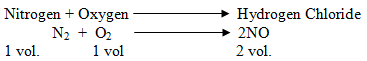## Latest Articles

In 1811 Amadeo Avogadro proposed his hypothesis. According to his hypothesis under the similar condition of temperature and pressure equal volume of all gases contain the same number of molecules.

i] To establish the relation between molecular weight and vapour density or,
Prove that: Molecular Weight = 2 × Vapour Density
Mol. Wt. = 2 × V.D.

Vapour density is the ratio of weight of certain volume of a gas to the weight of same volume of hydrogen under the similar condition of temperature and pressure.

ii] Elementary Gases are diatomic gases
a] Hydrogen is a diatomic gas.

n molecules                   n molecules                 2n molecules
1 molecule                    1 molecule                   2 molecules
1/2 molecule                 1/2 molecule                1 molecule

Hydrochloric Acid reacts with base to give only one type of salt. So one molecule of HCl contains only one atom of hydrogen which comes from 1/2 molecule of hydrogen.
Thus,
1/2 molecule of hydrogen = 1 atom of hydrogen
1 molecule of hydrogen = 2 atoms of hydrogen
Hence, Hydrogen is a diatomic gas.

b] Chlorine is a diatomic gas.

n molecules                   n molecules                 2n molecules
1 molecule                    1 molecule                   2 molecules
1/2 molecule                 1/2 molecule                1 molecule

Experimentally it has been found that molecular weight of HCl is 36.5 and it contains one atom of hydrogen. So weight of chlorine in a molecule of hydrogen chloride is 35.5. This weight is atomic weight of chlorine. So one molecule of HCl contains one atom of Chlorine which from 1/2 molecule of Chlorine.

Hence,
1/2 molecule of Chlorine = 1 atom of Chlorine
1 molecule of Chlorine = 2 atoms of Chlorine
Thus, Chlorine is a diatomic gas.

c] Nitrogen is a diatomic gas.n molecules                   n molecules                 2n molecules
1 molecule                    1 molecule                   2 molecules
1/2 molecule                 1/2 molecule                1 molecule

Experimentally it has found that molecular weight of Nitric Oxide is 30, and it contains one atom of oxygen. So weight of nitrogen in a molecule of nitric oxide is 14. This weight is atomic weight of nitrogen. So one molecule of nitric oxide contains one atom of nitrogen which comes from 1/2 molecule of nitrogen.
Hence,
1/2 molecule of nitrogen = 1 atom of nitrogen
1 molecule of nitrogen = 2 atoms of nitrogen
Thus, Nitrogen is a diatomic gas.

d] Oxygen is a diatomic gas:n molecules                   n molecules                 2n molecules
1 molecule                    1 molecule                   2 molecules
1/2 molecule                 1/2 molecule                1 molecule

Experimentally it has found that molecular weight of Nitric Oxide is 30 and it contains one atom of nitrogen. So weight of oxygen in a molecule of nitric acid is 16. This weight is atomic weight of oxygen. So one molecule of nitric oxide contains one atom of oxygen, which comes from 1/2 molecule of oxygen.
Hence,
1/2 molecule of oxygen = 1 atom of oxygen
1 molecule of oxygen    =  2 atoms of oxygen
Thus, oxygen is a diatomic gas.

iii] Molar Volume of each gas at STP is 22.4 litre
According to application of Avogadro's hypothesis
Molecular Weight = 2 × Vapour Density
or,